### The classification of finite simple groups

A group is a collection of elements that obey to certain rules. For every group we can construct some subgroups, in particular the normal subgroups. Given a group $G$, a subgroup $K$ is normal if, for every element $g \in G$ $gK = Kg$ or in a trivial way if every element $g \in G$ commute with every element $k \in K$.
Now, if the set of the normal subgroups of a nontrivial given group $G$ is constituted only by the trivial group and the group itself, then $G$ is a simple group. And if the group $G$ is finite (the number of the elements of the group is finite), so $G$ is a finite simple group.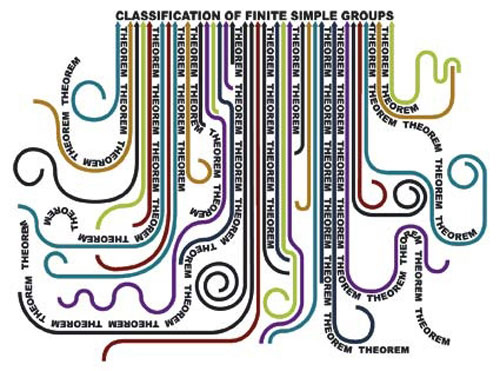In order to classify the finite simple groups, Daniel Gorenstein, Ron Solomon and Richard Lyons started in 1980s a program to produce a new and complete proof of the Classification theorem(1):
Every finite simple group is isomorphic to one of the following groups:
• A cyclic group with prime order;
• An alternating group of degree at least 5;
• A simple group of Lie type, including both
• the classical Lie groups, namely the groups of projective special linear, unitary, symplectic, or orthogonal transformations over a finite field;
• the exceptional and twisted groups of Lie type (including the Tits group which is not strictly a group of Lie type).
• The 26 sporadic simple groups.
The work was concluded by Michael Aschbacher and Stephen Smith in 2004: the last chapter of the proof was described in a not so technical paper only by Aschbacher(6) and after in two mathematical monographs(7). The complete classification was finally published in 2011 in the monograph The Classification of Finite Simple Groups: Groups of Characteristic 2 Type by Aschbacher, Lyons, Smith, and Solomon (Mathematical Surveys and Monographs, vol. 172). This work wins the 2012 Leroy P. Steele Prize for Mathematical Exposition(9):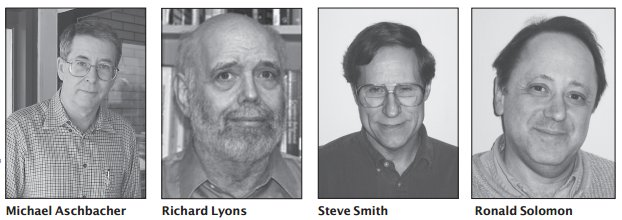In this paper, the authors, who have done foundational work in the classification of finite simple groups, offer to the general mathematical public an articulate and readable exposition of the classification of characteristic 2 type groups.

### MoonKAM: The Universe's First Student-Run Planetary CameraMoonKAM is an educational project developed by Nasa. Some students form a selected school (the Emily Dickinson Elementary School in Bozeman, Mont.) had the possibility to request moon's surface photos taken from twin satellites orbiting the Moon in order to map its gravitational field.
Some days ago, Nasa released the first images selected by students:

### There are the rushing waves

A scientific poetry by Richard Feynman from The value of science (pdf):
There are the rushing waves
mountains of molecules
each stupidly minding its own business
trillions apart
yet forming white surf in unison.
Ages on ages before any eyes could see
year after year
thunderously pounding the shore as now.
For whom, for what?
with no life to entertain.
Never at rest
tortured by energy
wasted prodigiously by the sun
poured into space.
A mite makes the sea roar.
Deep in the sea
all molecules repeat
the patterns of one another
till complex new ones are formed.
They make others like themselves
and a new dance starts.
Growing in size and complexity
living things
masses of atoms
DNA, protein
dancing a pattern ever more intricate.
onto dry land
here it is
standing:
atoms with consciousness;
matter with curiosity.
Stands at the sea,
wonders at wondering: I
a universe of atoms
an atom in the universe.
(via Amedeo Balbi)

### The difference between science and press release

Thanks to @peppeliberti for sharing the preprint.

Do you remember the famous OPERA preprint about superluminal neutrinos? After the publication of the results about neutrinos' flight time, a lot of preprint was published on arXiv in order to explain results. Some researchers try to explain with esperimental problems (and it could be the right explenation), some researchers propose their theoretical explenation, Andrew Cohen and Sheldon Glashow(1) reject results using some simple theoretical arguments:
superluminal neutrinos would lose energy rapidly via the bremsstrahlung of electron-positron pairs(1)
where bremmsstrahlung radiation is the radiation produced by the deceleration of a charged particle. In particular Cohen and Glashow studied the neutrinos' processes analog to Cherenkov radiation for neutral particle caused by weak interaction. Their results
cannot be reconciled with the claimed superluminal neutrino velocity measurement(1)
After a few days ICARUS experiment, based in LNGS (the same laboratories of OPERA), confirm the theoretical arguments discussed by Chen and Glashow:
No Cherenkov like event has been detected in ICARUS(2)
Now ICARUS, an experiment designed by Nobel Pirze Carlo Rubbia, upload a new preprint about superluminal neutrinos' saga: Measurement of the neutrino velocity with the ICARUS detector at the CNGS beam.
In this case, ICARUS, using the same beam send by CERN to OPERA from the 21st october to the 6th november 2011(3), measure the time of flight of neutrinos produced at CERN. In the preprint they describe also some experimental problems, but the collaboration resolve them, and publish they results: neutrinos fly at light speed (or a bit less), like you can see in the following plot(3):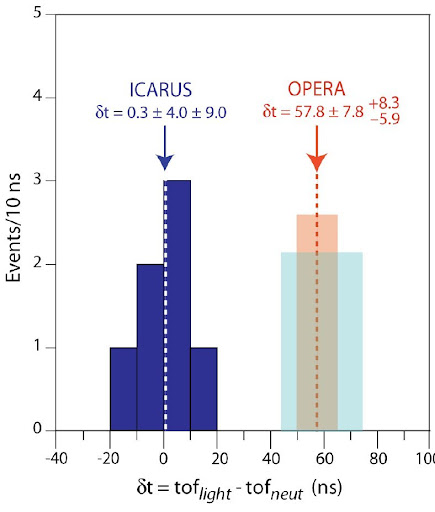Furthermore, ICARUS' preprint is written like an alternative to the previous OPERA's preprint: indeed they describe the synchronization between CERN and LNGS, using the same timing system of OPERA. I repeat againg: ICARUS, using the OPERA's GPS system, find $c$-neutrinos, and not superluminal neutrinos, so the OPERA's cable problem (the cause of superluminal data) is the most important source in OPERA's mistaken data.
So I think that here is the difference between science, represented by ICARUS, and press release, represented by OPERA: for science the most important thing is the knwoledge of nature, also if this is mean publish results after others; for press release is important to write also about coffe break.
(1) Andrew Cohen, Sheldon Glashow. New Constraints on Neutrino Velocities
(2) ICARUS collaboration. A search for the analogue to Cherenkov radiation by high energy neutrinos at superluminal speeds in ICARUS
(3) ICARUS collaboration. Measurement of the neutrino velocity with the ICARUS detector at the CNGS beam
Superluminal Saga: Waiting superluminal neutrinos (if they exist!) | Waiting superluminal neutrinos: from Maxwell to Einstein | Probably not | News from the OPERA | Experimental problems in OPERA

### Pi Day and the BBP formula

In the last few days I must write the 47th italian Carnival of Mathematics (Google translation), so I cannot write something about Pi Day. So, in the end of the day, I try to write a little post about this day.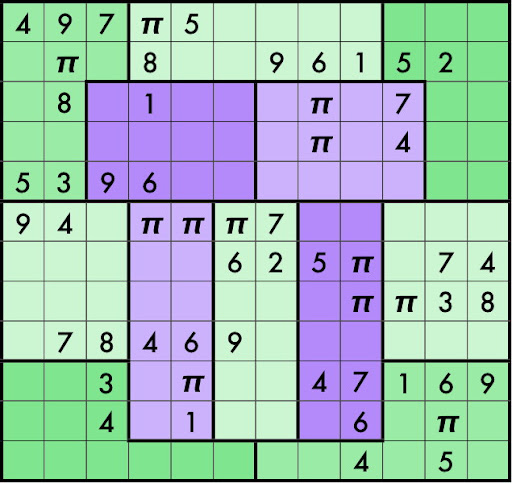$\pi$ is one of the irrational numbers: we cannot write an irrational numbers like a ratio between twointeger numbers, so Pitagora's team called them irrational and hidden their existence. We can define $\pi$ like the ratio between circle and his diameter, and it's impossible that they are contemporary integer. This assertion was proofed in 1761 by Johann Heinrich Lambert, while in 1882 Ferdinand von Lindemann proofed that $\pi$ is a trascendental number, where a trascendental number is a number that we cannot calculate with usual algebric operations.
In ancient times, the first mathematician who evaluate $\pi$ was Archimedes, who used the method of exhaustion, that is a method to evaluate the area of a shape by inscribing inside it a series of polygons that converge to area's shape. In this way Archimedes find $\pi = \frac{211875}{67441} = 3.14163 \cdots$
Today we know 5 triolions of digits, thanks to the work of Shigeru Kondo, who modify a usual home pc in order to perform the calculations using the softwerdeveloped by Alexander Yee. They design an internet site to describe methods, software and results.
Following David Bailey and Jonathan Borwein(1), I find some others interesting site about the calculations of $\pi$'s digits (Inverse Symbolic Calculator, Electronic Geometry Models). Other links at Experimental mathematics. But... what is experimental mathematics?
(...) namely the utilization of modern computer technology as an active tool in mathematical research.(1)
For example using the PSLQ algorithm(2) in 1995 was found the BBP formula for $\pi$: $\pi = \sum_{k=0}^\infty \frac{1}{16^k} \left ( \frac{4}{8k+1} - \frac{2}{8k+4} - \frac{1}{8k+5} - \frac{1}{8k+6} \right )$
This formula permits one to directly calculate binary or hexadecimal digits beginning at the $n$-th digit, without needing to calculate any of the first $n − 1$ digits, using a simple scheme that requires very little memory and no multiple-precision arithmetic software.(1)
You can find others BBP formulas in the David Bailey's compendium (pdf).

### The witch of Agnesi

The first woman known as mathematichian was the italian Maria Gaetana Agnesi (1718-1799) and hermost famous work was Instituzioni analitiche ad uso della gioventù italiana (Analytical institutions for italian young people), in which she studied an old famous curve callde versiera, in anglosaxon worlds the witch of Agnesi. The name derives from a mistranslation by John Colson who intend the original italian therm averisera, that means versed sine curve, like avversiera, that in english became witch or wife of devil.
The curve was studied for the first time by Pierre de Fermat in 1630, and after by Guido Grandi in 1703 and in 1748 by Maria in her treatise. The curve was defined by: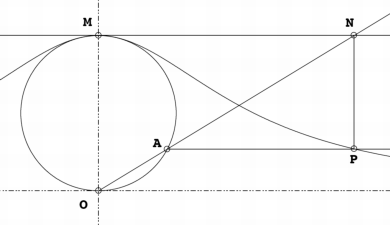(commons)
Starting with a fixed circle, a point $O$ on the circle is chosen. For any other point $A$ on the circle, the secant line $OA$ is drawn. The point $M$ is diametrically opposite $O$. The line $OA$ intersects the tangent of $M$ at the point $N$. The line parallel to $OM$ through $N$, and the line perpendicular to $OM$ through $A$ intersect at $P$. As the point $A$ is varied, the path of $P$ is the witch.
Its cartesian equation is $y = \frac{8a^3}{x^2 + 4a^2}$ In 1918 Frederick H. Hodge proofed that the witch is generated from the following curve

### Higgs' research: CDF and D0 confirm ATLAS and CMS results

Do you remember the conference of ATLAS and CMS about their Higgs' preliminary results? Well. Today during Moriond 2012 conference, the two Tevatron's collaboration, CDF and D0, presented their results about Higgs research: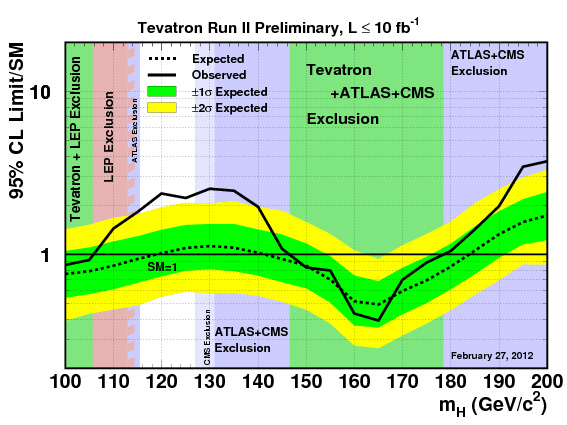(the plot shows the upper limit on the Higgs boson production rate)
In synthesis they confirm LHC's results (Tevatron's Higgs range: 115 GeV - 135 GeV, with $sigma = 2.2$).
More details on: Fermi Lab's press release, Tommaso Dorigo, the source of the image.
Special thanks to Peppe Liberti.